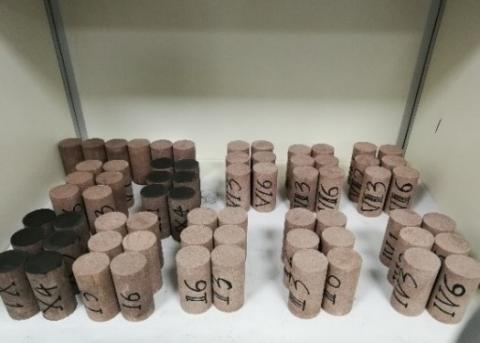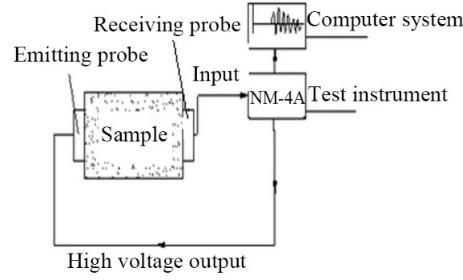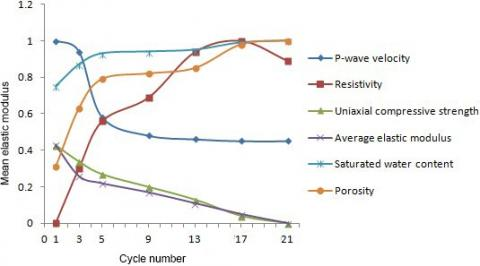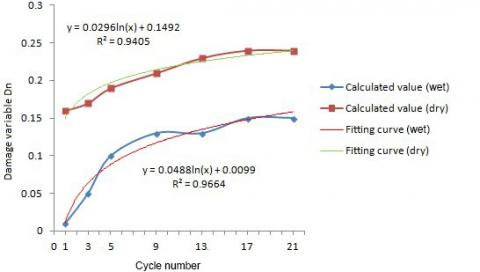# Evaluation of Cumulative Damage of Sandstone under Cyclic Wetting and Drying through Acoustic Wave Parameters and Resistivity Testing

Evaluation of Cumulative Damage of Sandstone under Cyclic Wetting and Drying through Acoustic Wave Parameters and Resistivity Testing
Hydraulic Building Health Diagnostic Technology Chongqing University Engineering Research Center, Chongqing Jiaotong University, Chongqing 400074, China

School of Civil Engineering, Hunan City University, Yiyang 413000, China

Corresponding Author Email:
990201300054@cqjtu.edu.cn
Page:
256-261
|
DOI:
https://doi.org/10.14447/jnmes.v23i4.a06
Received:
5 June 2020
|
Accepted:
2 October 2020
|
Published:
31 December 2020
| Citation
Abstract:

This paper carries out cyclic wetting and drying experiments on the sandstones from the bank slope of an inland port, and explores the deterioration features and acoustic wave parameters and resistivity (AWPR) of the sandstone. The variations in P-wave velocity, attenuation coefficient, nonlinear coefficient, resistivity, and other indices with the cycle number were discussed in details. Then, the correlations between P-wave velocity, resistivity, and physical-mechanical indices were analyzed based on the experimental results. Through the analysis on instantaneous damage analysis, a cumulative damage model was proposed, in the light of the AWPR. The results show that: the evolution of sandstone resistivity can accurately reflect the deterioration features of the sandstone under cyclic wetting and drying, providing a desirable tool to characterize rock strength deterioration and internal damage changes. Taking cycle number into account, the proposed theoretical model for cumulative damage can derive the damage and deterioration of sandstone excellently. By this model, the AWPR at any moment can be acquired in real time on site, which is convenient for engineering application.

Keywords:

sandstone, cyclic wetting and drying, P-wave velocity, acoustic wave parameters and resistivity (AWPR) testing, cumulative damage

1. Introduction

The boom of large water conservancy projects has amplified the annual variation in water level of inland ports, which complicates the occurrence environment of rock and soil masses under water. During port operations, special attention should be paid to the changes of cracking, damage, and stability of the rock mass on the bank slope.

Rock mass is a kind of porous medium material with complex internal structure. The macro-mechanical performance of the rock mass is often closely related to its internal pores and fissures. The degree of damage of the rock mass varies with the magnitude and type of external forces. The internal damage of the rock mass can be better identified based on the change of porosity [1-3].

The internal damage will accumulate faster under cyclic wetting and drying. Once cumulative damage reaches a certain threshold, the bank slope will become unsuitable for engineering application. Fu et al.  studied the evolution law of the microscopic damage in sandstone under cyclic wetting and drying through experiments.

With the rise and fall of water level, the rock mass of the bank slope is subject to cyclic wetting and drying, resulting in many complex internal cracks. However, it is impossible to directly capture the changes of internal cracks in the rock mass induced by cyclic wetting and drying, not to mention revealing the damage evolution or evaluating the long-term stability of the rock mass of the bank slope. This calls for effective and feasible means to measure the crack features in the rock mass.

The acoustic wave parameters (AWPs) of the rock mass provide a tool to measure the internal cracks of the rock mass. Guo et al.  evaluated the change law of elastic wave velocity in structural damage zone, and established the wave propagation equation for water-containing porous media. Some scholars  have analyzed the relationship between the physical properties and AWPs of the rock mass, and quantified the sensitivity of AWPs to porosity [6-8]. Some have calculated the instantaneous damage of fractured rock mass, in the light of nonlinear coefficients like the velocity of P-wave.

In addition to porosity, the water content in the rock mass of bank slope also changes continuously. Through experiments, Chen et al. , Fu et al.  and Zhang et al.  proved that the resistivity of the rock mass is highly sensitive to water content. As two geophysical methods, AWP testing and resistivity testing have the advantages of being non-destructive, convenient, fast, effective, and low-cost. Yang et al.  explored the relationship between acoustic wave velocity and rock resistivity in the wetting process, and confirmed the complementarity between AWP testing and resistivity testing. But few attempts have been made to analyze the dynamic change of the rock mass by combining AWP testing with resistivity testing.

This paper integrates AWP testing with resistivity testing into AWPR testing, and carries out AWPR experiments to investigate the change law of various indices (e.g. P-wave velocity, attenuation coefficient, nonlinear coefficient, and resistivity) under different cyclic wetting and drying conditions. Based on the measured instantaneous damages, a cumulative damage model of AWPR parameters was established, in the light of the cycle number and the initial damage. The calculation results of the model can accurately reflect the damage and deterioration of sandstone, laying a scientific basis for strength prediction of the rock mass and long-term stability analysis of the bank slope.

2. AWPR Experiments

2.1 Experiment design

The sandstones were collected from the bank slope of an inland port in the Three Gorges Reservoir. The initial physical properties of the rock samples were as follows: natural water content 0.82%, natural density 2.39 g/cm3, initial porosity 9.01%, and initial uniaxial compressive strength 62.34MPa. The samples were processed into cylinders with a diameter of 50mm and a height of 100mm, before being numbered and grouped. Figure 1 provides the photo of the prepared samples.

## 1.jpgFigure 1. The sandstone samples

The experimental environment was designed with n cycles of 24h vacuum wetting and 24h drying (n=0, 1, 3, 5, 9, 13, 17, and 21). Under different cycles of wetting and drying, the AWPs of the sandstone samples were measured by an NM-4A nonmetallic ultrasonic detector, including the amplitude of incident wave, the amplitude of emergent wave, and the mean wave velocity. In the meantime, the nonlinear features of the sandstone samples under cyclic wetting and drying were measured by a Rigol DG1022U waveform signal generator, a Tektronix TDS2012C digital storage oscilloscope, a YE5872A power amplifier, and a personal computer (PC) terminal.

After the AWPs were tested, the resistivities of the sandstone samples were measured by the dipole method under different cycles of wetting and drying. The electrodes were arranged symmetrically at both ends of each sample. To reduce the error, a standard resistance of 5,000Ω was connected in series in the test device. Multimeters 1 and 2 were used to collect the voltage of the standard resistance and the voltage difference between the M and N poles of the sample. Finally, the strength of each sandstone sample was measured in turn. Figure 2 illustrates the AWPR experimental system.

## 2a.jpg(a) The AWP testing circuit

## 2b.png(b) The AWP testing image

## 2c.png(c) The resistivity testing circuit

## 2d.png(d) The resistivity testing image

Figure 2. The AWPR test system

2.2 Results analysis

As per the above experimental design, the AWPR experiments were carried out with 6 samples as a group. The mean wave velocity and resistivity under each cycle number were computed. On this basis, the attenuation coefficient and the nonlinear coefficient can be respectively calculated by :

$\alpha=8.686 \operatorname{Ln}\left(A_{1} / A_{2}\right) /\left(X_{2}-X_{1}\right)$       (1)

$\beta_{0}=A_{2} / A_{1}^{2}$       (2)

where, A1 and A2 are the amplitudes of the incident and emergent waves, respectively (dB); X1=0 and X2=0.1 are the heights of the sample in wet and dry states, respectively (m); α is the attenuation coefficient (dB/m); β0 is the second-order nonlinear coefficient.

The mean values of P-wave velocity, nonlinear coefficient, resistivity, and attenuation coefficient of the wet samples under the excitation voltages of 250, 500, and 1,000V are recorded in Table 1.

Table 1. The mean values of measured indices of the samples in wet state

 Sample number Cycle number (n) P-wave velocity (km/s) Attenuation coefficient (dB/m) Nonlinear coefficient Resistivity (Ω·m) Excitation voltage 250V 500V 1,000V I 0 2.31 38.61 40.13 41.54 0.0077 730.67 II 1 2.813 50.4 50.73 51.03 0.0165 134.61 III 3 2.798 49.06 49.25 49.62 0.0381 143.9 IV 5 2.611 47.96 47.88 47.96 0.0425 152.19 V 9 2.56 46.85 47.29 47.78 0.0449 156.14 VI 13 2.549 46.61 46.68 47.1 0.0472 164.02 VII 17 2.543 46.3 46.37 46.65 0.0508 165.77 VIII 21 2.542 46.21 46.23 46.89 0.0651 162.47

The measured data in Table 1 were normalized by:

$y_{i}=\frac{x_{i}-\min _{1 \leq j \leq n}\left\{x_{j}\right\}}{\max _{1 \leq j \leq n}\left\{x_{j}\right\}-\min _{1 \leq j \leq n}\left\{x_{j}\right\}}$      (3)

Figure 3 shows the variation of the normalized parameter values with the cycle number.

As shown in Figure 3, as the sample changed from the natural state to the first wet state, the velocity and attenuation coefficient of P-wave both increased significantly. With the growing cycle number, the two parameters exhibited a downward trend. The decline was sharp in the first few cycles, and tended to be stable after 5 cycles. Basically the same change law was observed on the velocity and attenuation coefficient of P-wave. In addition, the attenuation coefficient was not greatly affected by the excitation voltage; the nonlinear coefficient underwent a rapid and sustained growth with the growing cycle number. After the initial wet state, the resistivity decreased significantly, and then increased continuously from the 1st to the peak in the 17th cycle, before entering a decline from the 17th cycle.

The mean values of measured indices of the samples in dry state are recorded in Table 2.

The measured data in Table 2 were normalized by formula (3). Then, the variation of the normalized parameter values with the cycle number is plotted as Figure 4.

As shown in Figure 4, the velocity and attenuation coefficient of P-wave varied with the cycle number in the dry state similarly as that in the wet state. From the natural state to the first wet state, both parameters increased significantly, and then continuously decreased. After the first dry state, the nonlinear coefficient increased at a slow pace from the 1st to 13th cycle, and plunged after the 13th cycle. Compared with that in natural state, the resistivity of the sandstone was large after drying: the resistivity increased slowly before the 5th cycle, surged up between the 5th and 13th cycles, and decreased again after the 13th cycle.

## 3.jpgFigure 3. The variation of the normalized parameter values with the cycle number (wet state)

## 4.jpgFigure 4. The variation of the normalized parameter values with the cycle number (dry state)

Table 2. The mean values of measured indices of the samples in dry state

 Sample Cycle number (n) P-wave velocity (km/s) Attenuation coefficient (dB/m) Nonlinear coefficient Resistivity (Ω·m) Excitation voltage 250V 500V 1000V I 0 2.31 38.61 40.13 41.54 0.0077 730.67 II 1 2.526 47.8 48.24 48.63 0.1065 3,279.23 III 3 2.504 46.5 46.79 47.01 0.1152 3,629.71 IV 5 2.483 45.19 45.28 45.61 0.1207 4,085.05 V 9 2.449 39.38 40.44 41.66 0.1232 10,917.09 VI 13 2.419 38.62 39.42 40.89 0.1253 14,069.08 VII 17 2.401 37.18 38.1 39.67 0.0033 10,426.88 VIII 21 2.396 33.24 33.5 34.85 0.002 9,086.89

2.3 Correlation analysis

The results of AWPR testing intuitively reflect the change of sandstone resistivity with the cycle number. But the accumulation of damage under cyclic wetting and drying is essentially caused by the changing porosity and water content of the samples. To quantify the relationship between the AWPs and physical properties, the variations of relevant physical-mechanical indices of the sandstones under cyclic wetting and drying are displayed in Table 3.

After the data in Table 3 were normalized, the relationship between sandstone resistivity and physical-mechanical indices can be plotted as Figure 5.

As shown in Figure 5, the P-wave velocity dropped significantly in the first few cycles. This trend corresponded well with that of uniaxial compressive strength and mean elastic modulus. By contrast, the resistivity increased significantly, bearing high resemblance with the trend of porosity and saturated water content. In the latter cycles, the velocity of P-wave gradually tended to be gentle, while the uniaxial compressive strength continued to decline. After the 17th cycle, the resistivity decreased from the peak level.

## 5.jpgFigure 5. The relationship between sandstone resistivity and physical-mechanical indices

Table 3. The variations of relevant physical-mechanical indices of the sandstones

 Cycle number (n) 0 1 3 5 9 13 17 21 P-wave velocity (km/s) 2.31 2.831 2.798 2.611 2.56 2.549 2.543 2.542 Resistivity (Ω·m) 730.67 134.61 143.9 152.19 156.14 164.02 165.77 162.47 Uniaxial compressive strength (MPa) 62.336 47.013 44.666 42.525 40.683 38.992 36.36 35.381 Average elastic modulus (GPa) 9.119 6.37 5.562 5.356 5.125 4.817 4.513 4.298 Saturated water content (%) 0.822 3.843 4.31 4.537 4.578 4.627 4.8 4.832 Porosity (%) 8.042 9.089 10.195 10.725 10.836 10.955 11.387 11.455
3. Calculation of Cumulative Damage

In engineering, the internal damage of the rock mass is a dynamic variable, rather than a definite value. Hence, this paper proposes a concept called cumulative damage (Dc) related to the cycle number. According to the experimental results, the accumulation of cracks in the sandstone has a good correlation with the sandstone resistivity, under cyclic wetting and drying. To disclose the macro-mechanical effects of the micro-crack changes in sandstone, the cumulative damage was characterized by the cycle number, P-wave velocity, and resistivity.

3.1 Theoretical basis

Considering the relationship between the effective area and the effective stress, Kachanov and Rabotnow  described the damage of materials with the damage factor D:

$\sigma /{{\sigma }_{ef}}={{A}_{ef}}/A=1-D$       (4)

where, σ is the nominal stress; σef is the effective stress; A is the instantaneous area; Aef is the effective area.

Since it is difficult to measure the area before and after material damage, Lemaitre put forward the strain equivalence hypothesis, which defines D with the elastic modulus E based on Hooke’s law:

$\sigma /{{\sigma }_{ef}}={{A}_{ef}}/A=1-D$       (5)

where, ED is the elastic modulus after the damage.

By the Lammy equation of motion in elastic mechanics, the relationship between the elastic constant and the P-wave velocity can be derived:

$E=\frac{{{V}_{p}}^{2}\rho (1+\mu )(1-2\mu )}{1-\mu }$         (6)

where, E is the elastic modulus; Vp is the P-wave velocity; ρ is the density of the sandstone; μ is Poisson’s ratio.

Substituting formula (6) into formula (5), the damage factor can be calculated from P-wave velocity by:

$D\text{=}1-{{\left( \frac{{{V}_{P}}}{{{V}_{Pf}}} \right)}^{2}}$       (7)

where, Vpf is the P-wave velocity of the intact sandstone.

3.2 Damage model

Each index of the sandstone is changing dynamically under cyclic wetting and drying. Let Vpn be the wave velocity measured at a cycle number n≥0. Then, formula (7) can be rewritten as:

${{D}_{n}}\text{=}1-{{\left( \frac{{{V}_{Pn}}}{{{V}_{Pf}}} \right)}^{2}}$       (8)

Taking any moment as the initial state, n=0, the initial damage factor D0 can be calculated by:

${{D}_{0}}\text{=}1-{{\left( \frac{{{V}_{Pn}}}{{{V}_{Pf}}} \right)}^{2}}\text{=}1-{{\left( \frac{{{V}_{P0}}}{{{V}_{Pf}}} \right)}^{2}}$       (9)

where, Vpn is the P-wave velocity after n cycles (m/s); Vp0 is the measured P-wave velocity in the natural state (m/s); Vpf is the P-wave velocity of intact sandstone (m/s).

According to the current standard, the Vpf of intact sandstone falls between 1,500 and 4,000m/s. Thus, the mid-value of Vpf is 2,750 m/s. Taking the natural state as the initial state, the instantaneous damage variable at n=0 is D0=0.29. Then, the instantaneous damage variable at each cycle number (n≥1) was calculated by formula (10). The damage variables in the first few cycles were obtained through interpolation. Then, the damage evolution based on P-wave velocity is shown in Figure 6.

## 6.jpgFigure 6. The damage evolution based on P-wave velocity

As shown in Figure 6, with the growing cycle number, the damage variables derived from P-wave velocity were similar in dry and wet conditions. In the same cycle, the damage of dry sandstone was more severe than that of wet sandstone, indicating that cracking is the main cause of rock damage. It also reflects that P-wave velocity is highly sensitive to fissures.

As can be seen from the fitting results, the calculated values of the instantaneous damage variables in all n cycles satisfied the logarithmic relationship: Dn=Aln(n)+B, n≥1, where A and B are fitting coefficients determined through statistical analysis of the samples.

Damage tends to accumulate over the time. Through AWP testing, the wave velocity at a certain moment can be measured, and used to deduce the instantaneous damage. To grasp the dynamic change under cyclic wetting and drying, a cumulative damage Dc model related to the cycle number was established:

${{D}_{c}}\text{=}{{D}_{0}}+k\cdot {{D}_{n}}$      (10)

where, k is the correction factor depending on the water content and porosity of the sandstone at a certain moment. As shown in Figure 4, the sandstone resistivity is well correlated with water content and porosity, and the AWPR testing method has good applicability. From the relationship between resistivity and porosity, the correction coefficient can be derived from the measured resistivity:

$k\text{=}\frac{2\text{+}\phi }{21-\phi }\text{=}\frac{{{K}_{\rho }}}{{{K}_{\rho M}}},\left( 1<k<\frac{1-{{D}_{0}}}{{{D}_{n}}} \right)$       (11)

where, Kρ is the measured resistivity; KρM is the solid phase resistivity of the sandstone; ϕ is the porosity of the sandstone.

To sum up, the cumulative damage can be obtained from sandstone resistivity as:

${{D}_{c}}\text{=}{{D}_{0}}+k\cdot {{D}_{n}}\text{=1}-{{\text{(}\frac{{{V}_{P0}}}{{{V}_{Pf}}}\text{)}}^{2}}+\frac{{{K}_{\rho }}}{{{K}_{\rho M}}}\cdot \left\{ A\cdot \ln (n)+B \right\}$      (12)

3.3 Correlation analysis

By the classical formula (4) of the damage variable, the elastic modulus in the natural state was taken as the initial value. Then, the damage of the sandstone under cyclic wetting and drying was analyzed based on the measured mean elastic modulus in Table 3.

Figure 7 compares the results of the classical formula with those of the cumulative damage model (10).

## 7.pngFigure 7. The comparison between the results of the classical formula with those of the proposed model

As shown in Figure 7, the proposed model agrees well with the classic formula in calculation results. To calculate cumulative damage, the state of the sandstone at any time as the initial state. Then, the cumulative damage can be predicted with one year as the cycle. Once the cumulative damage reaches a threshold, the sandstone will no longer be applicable in engineering. The threshold can be determined based on lithological features and design requirements. From the threshold, the maximum cycle number and bank slope stability can be obtained.

4. Conclusions

Considering the influence of periodically changing water level, this paper carries out AWPR experiments on the resistivity and internal damage accumulation of the sandstone in the bank slope of an inland port under cyclic wetting and drying. The main conclusions are as follows:

(1) With the growing cycle number, basically the same change law was observed on the velocity and attenuation coefficient of P-wave. The two parameters decreased significantly in the first few cycles, stabilized between the 9th and 17th cycles, and then declined from the peak in the 17th cycle. In addition, the attenuation coefficient was not greatly affected by the excitation voltage. Resistivity and nonlinear coefficient both exhibited a trend of rapid-slow-rapid changes.

(2) The resistivity corresponds well to the changes in saturated water content and porosity, and P-wave velocity corresponds well to the uniaxial compressive strength and mean elastic modulus. This reflects the complementarily between P-wave velocity and resistivity.

(3) Considering the cycle number n, a cumulative damage model was designed based on the AWPR testing results. The model makes it easy to acquire the AWPR at any moment, and proposed model agrees well with the classic formula in calculation results, laying the theoretical basis for predicting rock mass strength and analyzing long-term stability of bank slope.

Acknowledgment

The research in this manuscript is fund by National Natural Science Foundation of China (Grant No.: 51609027), Research Project of Social Science in Yiyang City (Grant No.: 2020YS055), Scientific Research Project of Hunan Provincial Department of Education (Grant No.: 20C0379). The experiments are supported by the Key Laboratory of Water Conservancy and Water Transportation of the Ministry of Education, Chongqing Jiaotong University. We appreciate any institutions and individuals that have provided support for this paper.

References

 Cao, W.G., Li, X., Liu, F. (2007). Discussion on strain softening damage constitutive model for fissured rock mass. Chinese Journal of Rock Mechanics and Engineering, 26(12): 2488-2494. https://doi.org/10.3321/j.issn:1000-6915.2007.12.014

 Li, K.G., Qin, Q.C., Yang, B.W., Zhang, X.Y., Guo, W., Wang, T. (2018). Study on constitutive model of fractured rock mass damage considering initial macroscopic and microscopic defects. Journal of Safety Science and Technology, 14(12): 90-96. https://doi.org/10.11731/j.issn.1673-193x.2018.12.014

 Zhou, J.H., Yang, K., Fang, K., Zhao, T.B., Qiu, D.W. (2019). Effect of fissure on mechanical and damage evolution characteristics of sandstone containing hole defect. Journal of Central South University (Science and Technology), 50(4): 968-975. https://doi.org/10.11817/j.issn.1672-7207.2019.04.026

 Fu, Y., Wang, Z.J., Liu, X.R., Yuan, W., Miao, L.L., Liu, J. (2017). Meso damage evolution characteristics and macro degradation of sandstone under wetting-drying cycles. Chinese Journal of Geotechnical Engineering, 12(4): 1653-1661. https://doi.org/10.11779/CJGE201709013

 Guo, S.H., Guo, Y.C. (2013). Structural damage identification and image based on theory of elastic wave velocity for porous medium. Journal of Central South University (Science and Technology), 44(10): 4208-4213.

 Rahmouni, A., Boulanouar, A., Boukalouch, M., Géraud, Y., Samaouali, A., Harnafi, M., Sebbani, J. (2013). Prediction of porosity and density of calcarenite rocks from P-wave velocity measurements. International Journal of Geosciences, 4(9): 1292-1299. http://dx.doi.org/10.4236/ijg.2013.49124

 Wang, J.G., Gao, Q.C., Wang, X.Y., Liu, J., Jiang, B., Wu, W. (2014). The experimental analysis on influencing factors of P-wave velocity in red sandstone, Advanced Materials Research, 1030: 1023-1028. https://doi.org/10.4028/www.scientific.net/AMR.1030-1032.1023

 Han, X.H., Xu, D.H., Guo, J.X., Yang, L., Wang, Z.L., Zhang, D., Luo, X.P., Nie, J.G., Li, H., Jiang, J.Y. (2018). Rock physics modeling for acoustic velocities of sandstone considering effects of cementation and compaction. Chinese Journal of Geophysics, 61(12): 5044-5051. https://doi.org/10.6038/cjg2018M0214

 Zhao, H., Li, X.P., Luo, Y., Dong, Q., Huang, J.H. (2017). Characteristics of elastic wave propagation in jointed rock mass and development of constitutive model by coupling macroscopic and mesoscopic damage. Rock and Soil Mechanics, 38(10): 2939-2948. https://doi.org/10.16285/j.rsm.2017.10.022

 Zhou, X., Fan, L., Wu, Z. (2017). Effects of microfracture on wave propagation through rock mass. International Journal of Geomechanics, 17(9): 04017072. https://doi.org/10.1061/(ASCE)GM.1943-5622.0000947

 Liu, T., Li, X., Zheng, Y., Meng, F., Song, D. (2020). Analysis of seismic waves propagating through an in situ stressed rock mass using a nonlinear model. International Journal of Geomechanics, 20(3): 1-14. https://doi.org/10.1061/(ASCE)GM.1943-5622.0001621

 Chen, F., Xiu, J.G., An, J.Z., Liao, C.T., Chen, D.Y. (2000). Research on dependence of resistivity changing anisotropy on micro-cracks extending in rock with experiment. Acta Seismologica Sinica, 22(3): 310-318. https://doi.org/10.3321/j.issn:0253-3782.2000.03.012

 Fu, Y.C., Zhang, W.Q., Liu, D.P. (2016). Research on rock failure and resistivity variation feature. Coal Technology, 35(4): 97-99. https://doi.org/10.13301/j.cnki.ct.2016.04.041

 Zhang, B., Zhu, T., Zhou, J.G. (2017). Experimental studies on the changes of rock resistivitu image and anisotropy. Acta Seismologica Sinica, 39(4): 478-494. https://doi.org/10.11939/jass.2017.04.004

 Yang, W.M., Song, J., Liu, B., Li, S.C., Xu, X.J., Liu, Z.Y., Niu, L.C. (2015). Experimental research on wave velocity and electrical resistivity of rock-like material during saturation process. Chinese Journal of Rock Mechanics and Engineering, 34(4): 703-712. https://doi.org/10.13722/j.cnki.jrme.2015.04.006

 Zhao, M.J. (2009). Acoustic Characteristics and Application of Loaded Rock Concrete. Beijing: Science Press.

 Xie, H.P. (1990). Rock Concrete Damage Mechanics. Xuzhou: China University of Mining and Technology Press.